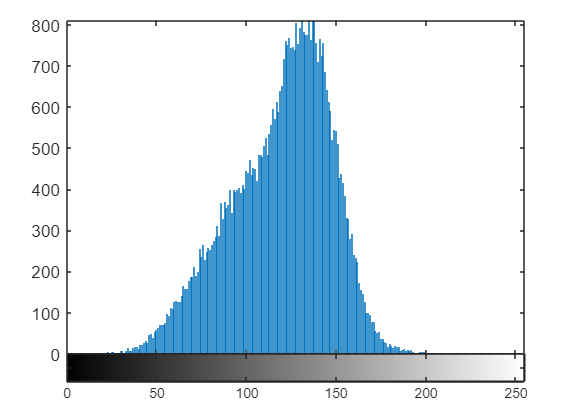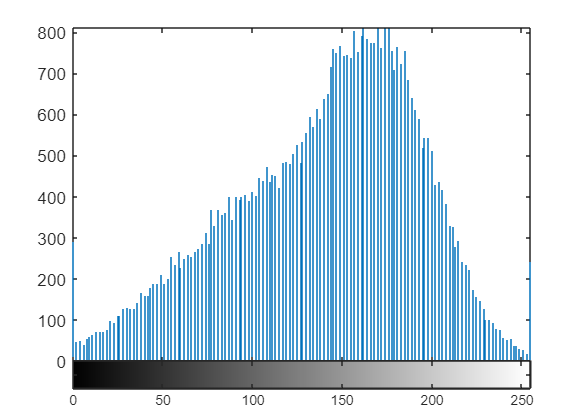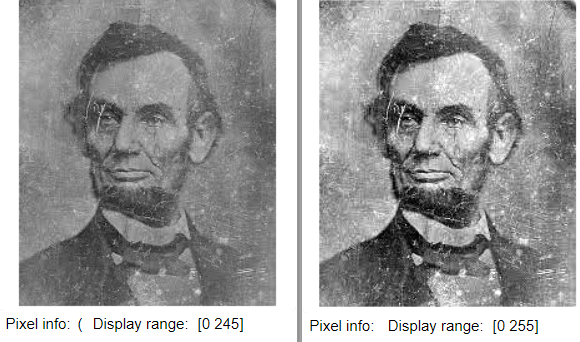Open in App
Not now

# How to Normalize a Histogram in MATLAB?

• Last Updated : 08 Dec, 2021

Histogram normalization is a technique to distribute the frequencies of the histogram over a wider range than the current range. This technique is used in image processing too. There we do histogram normalization for enhancing the contrast of poor contrasted images.

Formula:Here ./ and .* means operation has to be performed element-wise.

Steps:

• Convert color image into grayscale.
• Display histogram.
• Observe maximum and minimum intensities from the histogram.
• Change image type from uint8 to double.
• Apply a formula for histogram normalization.
• Convert back into unit format.
• Display image and modified histogram.

Example:

## Matlab

 `% MATLAB code for``% Histogram normalisation.``% Read the image.``k=imread(``"lincoln.jfif"``);` `% Convert into grayscale``k1=rgb2gray(k);` `% Display the image and histogram.``imtool(k1,[]);``imhist(k1);` `% Set the minimum and maximum``% Values from histogram.``min=45;``max=180;` `% Convert image into double.``k2=double(k1);` `% Apply the formula.``k3=(k2-min)./(max-min);` `% Multiply with maximum possible value.``k4=k3.*255;` `% Convert the final result into uint8.``k5=uint8(k4);` `% Display the enhanced image and histogram.``imtool(k5,[]);``imhist(k5);`

Output:Figure: Original histogramFigure: Normalized histogramFigure: Images before and after normalization

Code Explanation: